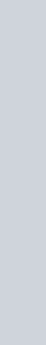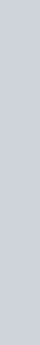# NCERT Solutions for class 11 maths chapter 1 Sets

## Exercise 1.1

Page No. 4

QUESTION 1:

(i) The collection of all the months of a year beginning with the letter J.
(ii) The collection of ten most talented writers of India.
(iii) A team of eleven best-cricket batsmen of the world.
(iv) The collection of all boys in your class.
(v) The collection of all natural numbers less than 100.
(vi) A collection of novels written by the writer Munshi Prem Chand.
(vii) The collection of all even integers.
(viii) The collection of questions in this Chapter.
(ix) A collection of most dangerous animals of the world.

SOLUTIONS:

(i) It is a Set.

Justification: One can definitely decide the months beginning with letter J, hence the collection of months of a year Beginning with J is a well-defined collection of objects.

(ii) It is not a Set.

Justification: For different persons, the ten most talented writers in India can be different. Because for one person a particular writer may be the most talented and for another person, he may not be the most talented. Hence, the collection will vary from person to person, and the collection will not be well defined.

(iii) It is not a Set.

Justification: For different persons, the eleven best batsmen of the world can be different. Because for one person a particular batsman may be the best and for another person, he may not be the best. Hence, the collection will vary from person to person, and the collection will not be well defined.

(iv) It is a Set.

Justification: The boys in my class are definite while making a collection one can definitely decide which boy should belong to the collection. Hence, the collection of all boys in my class is a well-defined collection of objects.

(v) It is a set.

Justification: The natural numbers less than 100 are definite while making a collection one can definitely decide what numbers should belong to the collection. Hence, the collection of all natural numbers less than 100 is a well-defined collection of objects.

(vi) It is a set.

Justification: The novels written by the writer Munshi Prem Chand are definite while making a collection one can definitely decide the novels which should belong to the collection. Hence, the collection of novels written by the writer Munshi Prem Chand is a well-defined collection of objects.

(vii) It is a set.

Justification: One can definitely decide the integers divisible by 2. Hence, the collection of all even integers is a well-defined collection of objects.

(viii) It is a set.

Justification: The questions in this Chapter are definite and one can definitely decide the questions which should belong to the collection. Hence, the collection of questions in this Chapter is a well-defined collection of objects.

(ix) It is not a set.

Justification: For different persons, the most dangerous animals of the world can be different. Because criteria for an animal to be called as dangerous can vary person to person. Some may consider dog as a dangerous animal while others may consider dog as a cute animal. Hence, the collection will not be well defined.

Page No. 5

Question 2:

Let A = {1, 2, 3, 4, 5, 6}. Insert the appropriate symbol ∈ or ∉ in the blank
spaces:
(i) 5. . .A (ii) 8 . . . A (iii) 0. . .A
(iv) 4. . . A (v) 2. . .A (vi) 10. . .A

Solution:

(i) 5 A

(ii) 8 A

(iii) 0 A

(iv) 4 A

(v) 2 A

(vi) 10 A

Question 3:

Write the following sets in roster form:
(i) A = {x : x is an integer and –3 ≤ x < 7}
(ii) B = {x : x is a natural number less than 6}
(iii) C = {x : x is a two-digit natural number such that the sum of its digits is 8}
(iv) D = {x : x is a prime number which is divisor of 60}
(v) E = The set of all letters in the word TRIGONOMETRY
(vi) F = The set of all letters in the word BETTER

Solutions:

(i) A = {x: x is an integer and –3 < x < 7}

exp: Integers greater than -3 and less than 7 are: –2, –1, 0, 1, 2, 3, 4, 5, and 6.

Thus elements of the set are –2, –1, 0, 1, 2, 3, 4, 5, 6.

Therefore, the given set can be written in roster form as

A = {–2, –1, 0, 1, 2, 3, 4, 5, 6}

(ii) B = {x: x is a natural number less than 6}

exp: Natural numbers less than 6 are: 1, 2, 3, 4, and 5.

Thus elements of the set are 1, 2, 3, 4, 5

Therefore, the given set can be written in roster form as

B = {1, 2, 3, 4, 5}

(iii) C = {x: x is a two-digit natural number such that the sum of its digits is 8}

exp: Two-digit natural number such that the sum of its digits is 8 are:17, 26, 35, 44, 53, 62, 71, 80

Thus the elements of this set are 17, 26, 35, 44, 53, 62, 71, 80.

Therefore, this set can be written in roster form as

C = {17, 26, 35, 44, 53, 62, 71, 80}

(iv) D = {x: x is a prime number which is a divisor of 60}

exp:∴60 = 2 × 2 × 3 × 5

The elements of this set are 2, 3, and 5 only.

Therefore, this set can be written in roster form as D = {2, 3, 5}.

(v) E = The set of all letters in the word TRIGONOMETRY

exp: Letters in the word TRIGONOMETRY are: T R I G O N O M E T R Y

There are 12 letters out of which letters T, R, and O are repeated.

Thus elements of the set are T, R, I, G, O, N, O, M, E, T, R, Y

Therefore, the given set can be written in roster form as

E = {T, R, I, G, O, N, M, E, Y}

(vi) F = The set of all letters in the word BETTER

exp: Letters in the word BETTER are: B E T T E R

There are 6 letters in the word BETTER, out of which letters E and T are repeated.

Thus elements of the set are B, E, T, R

Therefore, this set can be written in roster form as

F = {B, E, T, R}

Question 4:

Write the following sets in the set-builder form :
(i) (3, 6, 9, 12} (ii) {2,4,8,16,32} (iii) {5, 25, 125, 625}
(iv) {2, 4, 6, . . .} (v) {1,4,9, . . .,100}

Solution:

(i) A= {x: x = 3n, where n ∈ N and n ≤ 4}

exp: It is evident from the elements that 3=3×1, 6=3×2, 9=3×3, 12=3×4.

(ii) B ={ x: x = 2n, where n ∈ N and n ≤ 5}

exp: It is evident from the elements that 2=21, 4=22, 8=23, 16=24, 32=25

(iii) C ={ x: x =5n, where n ∈ N and n ≤ 4}

exp: It is evident from the elements that 5=51, 25=52, 125=53, 625=54

(iv) D ={x: x = 2n, where n ∈ N}

exp: It is evident from the elements that 2=2×1, 4=2×2, 6=2×3,…..

(v) E = { x: x = n2, where n ∈ N and n ≤ 10 }

exp: It is evident from the elements that 1=12, 4=22, 9=32,…, 100=102

Question 5.

List all the elements of the following sets :
(i) A = {
x : x is an odd natural number}
(ii) B = {
x : x is an integer,}
(iii) C = {
x : x is an integer, x2 4}
(iv) D = {
x : x is a letter in the word “LOYAL”}
(v) E = {
x : x is a month of a year not having 31 days}
(vi) F = {
x : x is a consonant in the English alphabet which precedes k }

Solution:

(i) A = {x: x is an odd natural number}

A= {1, 3, 5, 7, 9 …}

(ii) B = {x: x is an integer;}

exp: It can be seen thatand. Thus integers greater than 0.5 and less than 4.5 are: 0, 1, 2, 3, 4

B ={0, 1, 2, 3, 4}

(iii) C = {x : x is an integer, x2 ≤ 4}

exp: Integers whose squares are less than and equal to 4 are (-22)=4, (-1)=1, 02=0, 12=1, 22=4
The elements of the set are -2, -1, 0, 1, 2

C ={-2, -1, 0, 1, 2}

(iv) D = {x : x is a letter in the word “LOYAL”}

D = {L, O, Y, A}

(v) E = {x : x is a month of a year not having 31 days}

E = {February, April, June, September, November}

(vi) F = {x : x is a consonant in the English alphabet which precedes k }

F = {b, c, d, f, g, h, j}

Question 6

Match each of the set on the left in the roster form with the same set on the right described in set-builder form:
(i) {1, 2, 3, 6}                      (a) {x : x is a prime number and a divisor of 6}
(ii) {2, 3}                            (b) {x : x is an odd natural number less than 10}
(iii) {M,A,T,H,E,I,C,S}       (c) {x : x is natural number and divisor of 6}
(iv) {1, 3, 5, 7, 9}                (d) {x : x is a letter of the word MATHEMATICS}.

Solution: